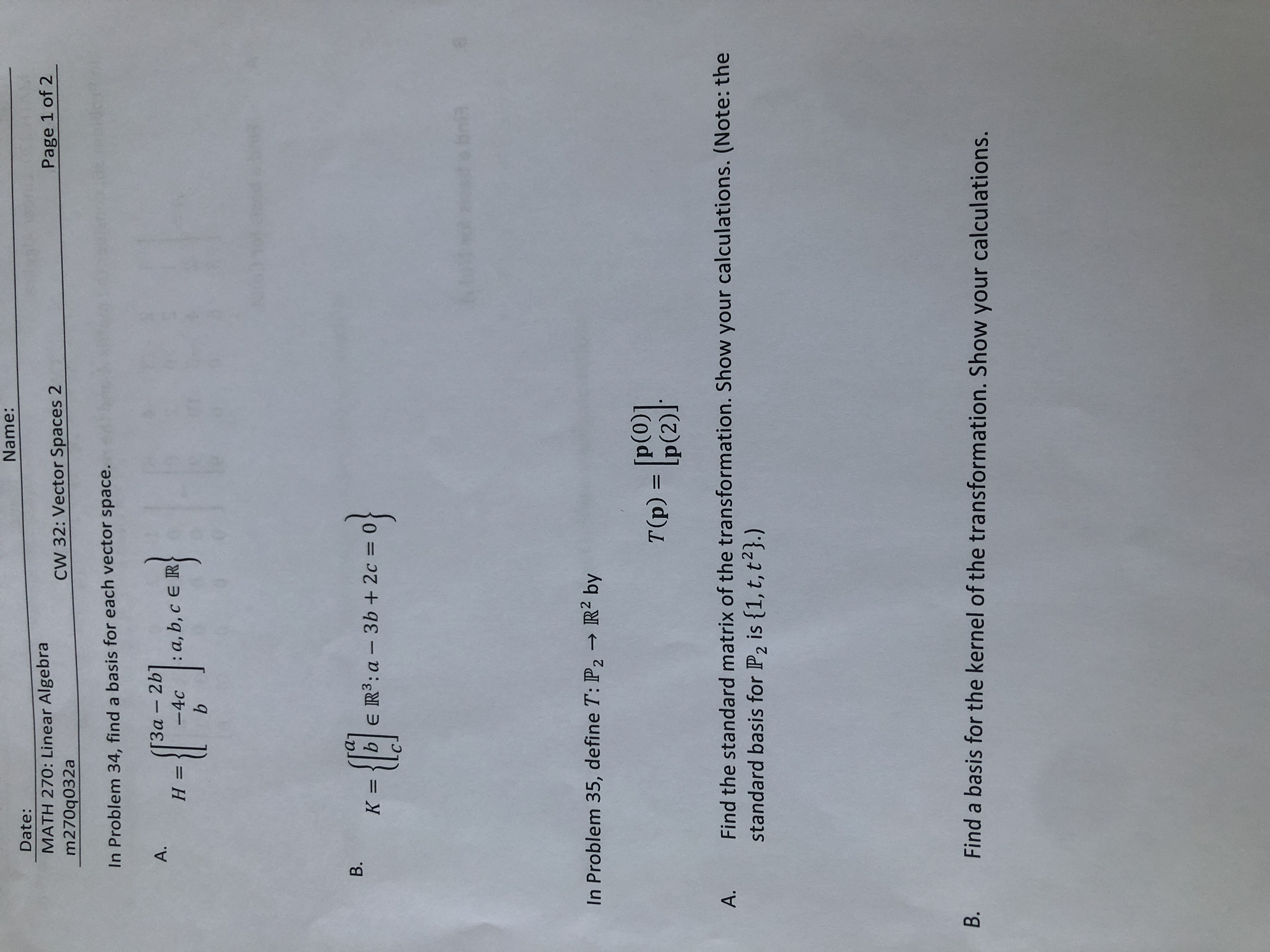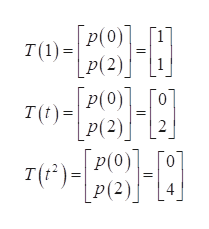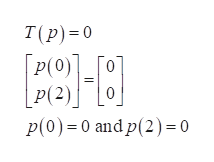Name:Date:MATH 270: Linear Algebram270q032aPage 1 of 2CW 32: Vector Spaces 2In Problem 34, find a basis for each vector space.heen3α-2b]-4c a, b, c E RA.H =bB.K =|bE R3:a3b + 2c=useobrinIn Problem 35, define T: P2R2 byp(0)]T(p) = Ip(2)Find the standard matrix of the transformation. Show your calculations. (Note: thestandard basis for P2 is {1, t, t2}.)A.Find a basis for the kernel of the transformation. Show your calculations.B.

Question

Vector spaces 2 Pratice not test all of ithelp_outlineImage TranscriptioncloseName: Date: MATH 270: Linear Algebra m270q032a Page 1 of 2 CW 32: Vector Spaces 2 In Problem 34, find a basis for each vector space. heen 3α-2b] -4c a, b, c E R A. H = b B. K =|b E R3:a 3b + 2c= useobrin In Problem 35, define T: P2R2 by p(0)] T(p) = Ip(2) Find the standard matrix of the transformation. Show your calculations. (Note: the standard basis for P2 is {1, t, t2}.) A. Find a basis for the kernel of the transformation. Show your calculations. B. fullscreen
Step 1

35

A

Obtain the standard matrix of the transformation as follows.

Given that standard basis for P2 is {1, t, t2}.help_outlineImage TranscriptioncloseГр(о]. p(0) 1 т1):| p(2)) Р(2) [Р(О1 Го T()p(2)2 Р (2) Tрe)- P(0)] Го P(2) 4 Т fullscreen
Step 2

The standard matrix of the transformation is

Step 3

B

Obtain the basis for the kernel of the transformation as follows.

Note that, the kernel of a linear transfo...help_outlineImage TranscriptioncloseT(p) 0 P(0)]_[O P(2)0 P(0) 0 andp(2) = 0 fullscreen

Want to see the full answer?

See Solution

Want to see this answer and more?

Our solutions are written by experts, many with advanced degrees, and available 24/7

See Solution
Tagged in

Math